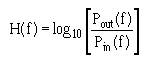Custom SearchDispersion reduces the bandwidth, or information-carrying capacity, of an optical fiber. Dispersion causes the spreading of the light pulse as it travels along the fiber (see figure 2-20). Fiber dispersion mechanisms include intramodal (chromatic) dispersion and intermodal (modal) dispersion. Multimode fiber bandwidth is a measure of the intermodal dispersion of the multimode fiber. Intermodal dispersion is maximum when all fiber modes are excited. The source used for intermodal dispersion measurements must overfill the fiber. The optical source must also have a narrow spectral width to reduce the effects of chromatic dispersion in the measurement. There are two basic techniques for measuring the modal bandwidth of an optical fiber. The first technique characterizes dispersion by measuring the impulse response h(t) of the fiber in the time domain. The second technique characterizes modal dispersion by measuring the baseband Frequency response H( f) of the fiber in the frequency domain. H(f) is the power transfer function of the fiber at the baseband frequency (f). H(f) is also the Fourier transform of the power impulse response h(t). Only the Frequency response method is described here. The test method for measuring the bandwidth of multimode fibers in the frequency domain is EIA/TIA-455-30. Signals of varying frequencies (f) are launched into the test fiber and the power exiting the fiber at the launched fundamental frequency measured. This optical output power is denoted as Pout( f). The test fiber is then cut back or replaced with a short length of fiber of the same type. Signals of the same frequency are launched into the cut-back fiber and the power exiting the cut-back fiber at the launched fundamental frequency measured. The optical power exiting the cutback or replacement fiber is denoted as Pin(f). The magnitude of the optical fiber Frequency response isThe fiber bandwidth is defined as the lowest frequency at which the magnitude of the fiber Frequency response has decreased to one-half its zero-frequency value. This is the -3 decibel (dB) optical power frequency (f3dB). This frequency is referred to as the fiber bandwidth. Bandwidth is normally given in units of megahertz-kilometers (MHz-km). Converting the -3 dB fiber bandwidth to a unit length assists in the analysis and comparison of optical fiber performance. For long lengths of fiber (>1km), the method for normalization is to multiply the length times the measured bandwidth. Q.9 The cutoff wavelength of matched-clad and depressed-clad single mode fibers varies according to the fiber's radius of curvature and length. The cutoff wavelength of which single mode fiber type is more sensitive to length? Q.10 Will the cutoff wavelength of uncabled fibers (λ cf) generally have a value higher or lower than the cutoff wavelength of cabled fibers (λcc)? Q.11 Describe the -3 decibel (dB) optical power frequency (f3dB).Integrated Publishing, Inc. - A (SDVOSB) Service Disabled Veteran Owned Small Business